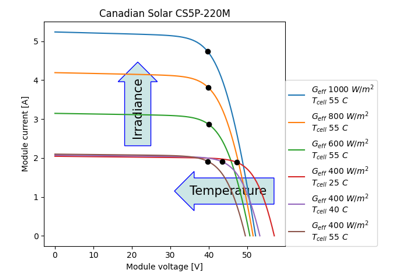# pvlib.pvsystem.singlediode¶

pvlib.pvsystem.singlediode(photocurrent, saturation_current, resistance_series, resistance_shunt, nNsVth, ivcurve_pnts=None, method='lambertw')[source]

Solve the single-diode equation to obtain a photovoltaic IV curve.

Solves the single diode equation 1

$I = I_L - I_0 \left[ \exp \left(\frac{V+I R_s}{n N_s V_{th}} \right)-1 \right] - \frac{V + I R_s}{R_{sh}}$

for $$I$$ and $$V$$ when given $$I_L, I_0, R_s, R_{sh},$$ and $$n N_s V_{th}$$ which are described later. Returns a DataFrame which contains the 5 points on the I-V curve specified in 3. If all $$I_L, I_0, R_s, R_{sh},$$ and $$n N_s V_{th}$$ are scalar, a single curve is returned, if any are Series (of the same length), multiple IV curves are calculated.

The input parameters can be calculated from meteorological data using a function for a single diode model, e.g., calcparams_desoto().

Parameters
• photocurrent (numeric) – Light-generated current $$I_L$$ (photocurrent) 0 <= photocurrent. [A]

• saturation_current (numeric) – Diode saturation $$I_0$$ current under desired IV curve conditions. 0 < saturation_current. [A]

• resistance_series (numeric) – Series resistance $$R_s$$ under desired IV curve conditions. 0 <= resistance_series < numpy.inf. [ohm]

• resistance_shunt (numeric) – Shunt resistance $$R_{sh}$$ under desired IV curve conditions. 0 < resistance_shunt <= numpy.inf. [ohm]

• nNsVth (numeric) – The product of three components: 1) the usual diode ideality factor $$n$$, 2) the number of cells in series $$N_s$$, and 3) the cell thermal voltage $$V_{th}$$. The thermal voltage of the cell (in volts) may be calculated as $$k_B T_c / q$$, where $$k_B$$ is Boltzmann’s constant (J/K), $$T_c$$ is the temperature of the p-n junction in Kelvin, and $$q$$ is the charge of an electron (coulombs). 0 < nNsVth. [V]

• ivcurve_pnts (None or int, default None) – Number of points in the desired IV curve. If None or 0, no points on the IV curves will be produced.

• method (str, default 'lambertw') – Determines the method used to calculate points on the IV curve. The options are 'lambertw', 'newton', or 'brentq'.

Returns

• OrderedDict or DataFrame

• The returned dict-like object always contains the keys/columns

• i_sc - short circuit current in amperes.

• v_oc - open circuit voltage in volts.

• i_mp - current at maximum power point in amperes.

• v_mp - voltage at maximum power point in volts.

• p_mp - power at maximum power point in watts.

• i_x - current, in amperes, at v = 0.5*v_oc.

• i_xx - current, in amperes, at V = 0.5*(v_oc+v_mp).

• If ivcurve_pnts is greater than 0, the output dictionary will also

• include the keys

• i - IV curve current in amperes.

• v - IV curve voltage in volts.

• The output will be an OrderedDict if photocurrent is a scalar,

• array, or ivcurve_pnts is not None.

• The output will be a DataFrame if photocurrent is a Series and

• ivcurve_pnts is None.

Notes

If the method is 'lambertw' then the solution employed to solve the implicit diode equation utilizes the Lambert W function to obtain an explicit function of $$V=f(I)$$ and $$I=f(V)$$ as shown in 2.

If the method is 'newton' then the root-finding Newton-Raphson method is used. It should be safe for well behaved IV-curves, but the 'brentq' method is recommended for reliability.

If the method is 'brentq' then Brent’s bisection search method is used that guarantees convergence by bounding the voltage between zero and open-circuit.

If the method is either 'newton' or 'brentq' and ivcurve_pnts are indicated, then pvlib.singlediode.bishop88() 4 is used to calculate the points on the IV curve points at diode voltages from zero to open-circuit voltage with a log spacing that gets closer as voltage increases. If the method is 'lambertw' then the calculated points on the IV curve are linearly spaced.

References

1

S.R. Wenham, M.A. Green, M.E. Watt, “Applied Photovoltaics” ISBN 0 86758 909 4

2

A. Jain, A. Kapoor, “Exact analytical solutions of the parameters of real solar cells using Lambert W-function”, Solar Energy Materials and Solar Cells, 81 (2004) 269-277.

3

D. King et al, “Sandia Photovoltaic Array Performance Model”, SAND2004-3535, Sandia National Laboratories, Albuquerque, NM

4

“Computer simulation of the effects of electrical mismatches in photovoltaic cell interconnection circuits” JW Bishop, Solar Cell (1988) https://doi.org/10.1016/0379-6787(88)90059-2

## Examples using pvlib.pvsystem.singlediode¶